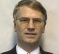## phase error correctionStarted by 2 years ago4 replieslatest reply 2 years ago179 views

Dear All,

I am working on the phase correction step in a receiver. The following algorithm has been already implemented:

• Â correct vectors (samples) by using the multiplication by exp (-1i * phI)
• Â compute an amplitude and rotate a vector:

A = sqrt(real^2+imag^2);

theta = arctan(imag/real);

• nowÂ theta will be the next angle for rotation

Actually, it is the standard algorithm to correct a phase, I think.

The simulation works but it gives me a big error (for me)\" dPhi\' (difference between phases adjacent samples) under low snr

Is there an algorithm to minimize the phase difference of two vectors??

[ - ]Are you simply averaging the theta values, which have roll-over, as that roll over can cause a significant non-linearity.

You could average the complex phase (i.e. the original complex signal divided by its amplitude) and then do the arctan step.

There are a range of choices depending on how you handle the varying amplitude.

Definitely worth some experimentation to see what is going on for the complex phase addition scenarios.

[ - ]i have tried...''the original complex signal divided by its amplitude''

it doesnt give me improvement

[ - ]the phase correction is done in freq domain, the timing correction is done in time domain, isnt?
[ - ]